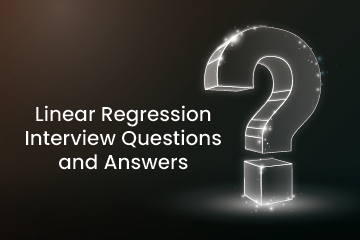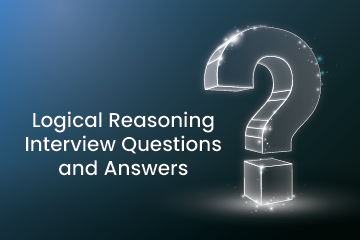Home / Blog / Interview Questions / Linear Regression Interview questions and Answers

# Linear Regression Interview questions and Answers

• October 29, 2022
• 3129
• 91### Meet the Author : Mr. Bharani Kumar

Bharani Kumar Depuru is a well known IT personality from Hyderabad. He is the Founder and Director of Innodatatics Pvt Ltd and 360DigiTMG. Bharani Kumar is an IIT and ISB alumni with more than 17 years of experience, he held prominent positions in the IT elites like HSBC, ITC Infotech, Infosys, and Deloitte. He is a prevalent IT consultant specializing in Industrial Revolution 4.0 implementation, Data Analytics practice setup, Artificial Intelligence, Big Data Analytics, Industrial IoT, Business Intelligence and Business Management. Bharani Kumar is also the chief trainer at 360DigiTMG with more than Ten years of experience and has been making the IT transition journey easy for his students. 360DigiTMG is at the forefront of delivering quality education, thereby bridging the gap between academia and industry.

• ### Is it possible that by the transformation the R SQR value increases so does the RMSE value`

• a) Not possible in Simple linear but possible in Multi linear
• b) Not Possible
• c) Possible
• d) Not possible in Multilinear but possible in Simple linear

When the data is transformed the intention is to improve the Correlation between the Features and the target. But one of the side effects of this can be that the transformation may increase the correlation but also increase the Error. It may be noted that R2 is a measure of a combination of 2 measures the SSR and the SSE. The SSR measures how much the inputs contribute to the change in the output. In the perspective of simple linear regression, this can be understood as the slope of the model vis-a-vis the baseline. The SSE measures the squared errors ie the difference between the actual and predicted squared. Sometimes after the transformation, the model SSR may increase excessively while the SSE also increases but not to that extent. Therefore the overall R2 may increase but the offset is that RMSE (due to the increased SSE) will also increase

• ### Which of the following is correct about Heteroscedasticity?

• a) The variance of the errors is not constant
• b) The variance of the dependent variable is not constant
• c) The errors are not linearly independent of one another
• d) The errors have non-zero mean

Answer - a) The variance of the errors is not constant

Explanation: The term heteroscedasticity will be called when the variance of the errors is not constant and following high and low error variance or following some patterns like funnel shape is called heteroscedasticity. A residual plot can help us to understand this scenario. Calculate Square residuals and plot the graph by taking squared residuals against the explanatory variable. If the scatterplot plotted between dependent and independent variables are varying in magnitude we can understand this may lead to unequal variances. If this problem exists, the population used in the regression contains unequal variance, and the analysis results may be invalid. To fix this problem we can perform transformations.

• ### Which of these points reflect the assumption of multicollinearity?

• a) There must not be any extreme scores in the data set
• b) An independent variable cannot be a combination of other independent variables
• c) The variance across the variables must be equal
• d) The relationship between your independent variables must not be above r = 0.7

Answer - d) The relationship between your independent variables must not be above r = 0.7

Multicollinearity refers to a situation in which two or more explanatory variables in a multiple regression model are highly linearly related. HDL and LDL are independent variables of the Regression technique. This is an example of perfect collinearity.MultiCollinearity is caused because of the inaccurate use of dummy variables. Multicollinearity generates a high variance of the estimated coefficients so the results will not be accurate. This problem will not allow for the extraction of the individual effects of each independent variable on the target variable. Due to this standard errors may be overestimated and t values are depressed. It can be detected through the Variance Inflation Factor.

• ### Variance inflation factor is used to regulate_________

• a) Multicollinearity
• b) Estimating regression coefficients
• c) both
• d) none of the above

A variance inflation factor (VIF) provides a measure of multicollinearity among the independent variables in a multiple regression model.Variance inflation factors allow a quick measure of how much a variable is contributing to the standard error in the regression.It measures how much the variance (or standard error) of the estimated regression coefficient is inflated due to collinearity.VIF=1/tolerance (1/1-Rˆ 2)and VIF is 1 indicates two variables are not correlated if it is >10 it is highly correlated.Due to the variance , the interpretation is difficult with respect to coefficients due to multicollinearity problem.the VIF for a regression model variable is equal to the ratio of the overall model variance to the variance of a model that includes only that single independent variable.

• ### The best evaluation metric for linear regression is____ ?

• a) RMSE
• b) MAE
• c) ME
• d) All the above

Absolute Error is the amount of deviation in our calculations. It is the difference between the predicted and the actual value. The Mean Absolute Error(MAE) is the mean of all absolute errors. RMSE is best as it is differentiable everywhere. To optimize the squared error, we can determine the derivative and set its expression equal to 0, and solve. But to optimize the absolute error, we require more complex techniques having more calculations. We use the Root Mean Squared Error instead of the Mean squared error so that the unit of RMSE and the dependent variable are equal and the results are interpretable. Mean Absolute Error(MAE) is preferred when we have too many outliers present in the dataset because MAE is robust to outliers whereas MSE and RMSE are very liable to outliers and these start reducing the outliers by squaring the error terms, commonly known as residuals.

• ### which of the following is not an assumption of linear regression?

• a) There should be linear and additive relationship between input and output
• b) There should be no correlation between residual terms
• c) Inputs should not be correlated
• d) Error terms should not be normally distributed

Answer - d) Error terms should not be normally distributed

Regression is a parametric approach and it makes assumptions for analysis. If the assumptions are not satisfied the results are not fruitful. There should be a linear relationship between x and y i.e 1unit change in x will have a change in y. An additive relationship is the effect of one input on y is independent of other variables in the data. If errors have a relationship we end up with an autocorrelation problem. If the inputs have strong relationships among them it is a multicollinearity problem. Errors should be normally distributed and should have constant variance. If the error terms are non-normally distributed, confidence intervals may become too wide or narrow.